Friday , August 19 2022# NCERT 5th Class (CBSE) Mathematics: Fractions

## RECIPROCALS

Two numbers whose product is 1 are called reciprocals.

For example, 5/6 and 6/5 are reciprocals.

5/6×6/5 = 30/30 = 1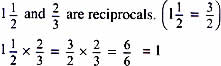4 and 1/4 are reciprocals.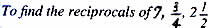Step 1. Write the number as a fraction             7/1, 3/4, 5/2

Step 2. Interchange the numerator and denominator    1/7, 4/3, 2/5

## DIVISION OF FRACTIONS

### Dividing Fractions

To understand division of fractions let us look at division again.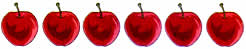If you had a sum 6÷2, you could think of it as ‘how many 2s are there in 6?’ The answer would be 3.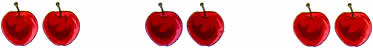There are three 2s in 6.

### Dividing a Whole Number by an Unit Fraction

(a) You can do the same thing when you divide by fractions.

To understand 3÷1/2, ask the question ‘how many halves in 3?’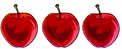These are three apples. Think: ‘how many halves in three apples?’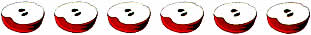We can see that there are 6 halves in 3 apples.

3÷1/2 = 6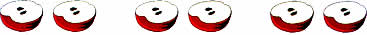We can also describe the same picture as 3×2 = 6

Dividing by 1/2 is just like multiplying by 2.

Here is how you can solve the sum without pictures.

3             ÷             1/2               = ?

Dividend                    Divisor

Step 1. Find the reciprocal of the divisor. Reciprocal of 1/2 is 2/1.

Step 2. Multiply the dividend with the reciprocal: 3×2/1 = 6
3÷1/2 = 6

## चुनौती हिमालय की 5th NCERT CBSE Hindi Rimjhim Ch 18

चुनौती हिमालय की 5th Class NCERT CBSE Hindi Book Rimjhim Chapter 18 प्रश्न: लद्दाख जम्मू-कश्मीर राज्य में …

### One comment

1.I need worksheet for Class 5th Mathematics.